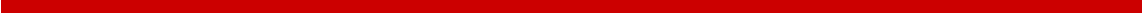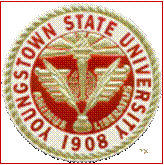Mathematical Models and Applications In general, a mathematical model is a description of a system using mathematical concepts and language. It can take many forms such as dynamical systems, statistical models, differential equations, game theoretic models, or stochastic process. I am  interesting in applications of mathematical models in finance, statistics, climate change, and actuarial sciences. Some of my favorite models are: Hidden Markov Models (HMM) In many real-world problems, the states of a system can be modeled as a Markov chain in which each state depends upon the previous state in a non-deterministic way. In hidden Markov models (HMMs), these states are invisible while observations (the inputs of the model), which depend on the states, are visible. I applied the model to predict economic regimes, stock prices, and weather conditions. Variance Gamma Model    Variance gamma (VG) process was introduced by Madan and Seneta in 1990 as a model for option pricing. The improvement in the VG model is that there is no continuous martingale component, allowing it to overcome the well-known shortcomings of the Black-Scholes model. Monte Carlo and Quasi Monte Carlo Method The Monte Carlo simulation is a popular numerical method across sciences, engineering, statistics, and computational mathematics. In simple terms, the method involves solving a problem by simulating the underlying model using pseudorandom numbers, and then estimates the quantity of interest as a result of the simulation. In recent years, a deterministic version of the MC method, the so-called quasi-Monte Carlo (QMC) method, has been widely used by researchers. Different from the MC method which relies upon pseudorandom numbers, QMC method uses low-discrepancy sequences for the sampling procedure. The QMC method converges generally faster than the corresponding MC method. One example of my research in this field is Acceptance-Rejection QMC Currently, the main method for transforming low-discrepancy sequences to non-uniform distributions is the inverse transformation technique. However, this technique can be computationally expensive for complicated distributions. The acceptance-rejection technique was developed precisely for this reason for the pseudorandom sequences. Research funding SOA individual research funding in finance: July 2016-June 2017, “Hidden Markov Model for Portfolio Management with Mortgage Backed Securities Exchange-Traded Funds”.
 Research Interests

## Nguyet (Moon) Nguyen

Assistant Professor